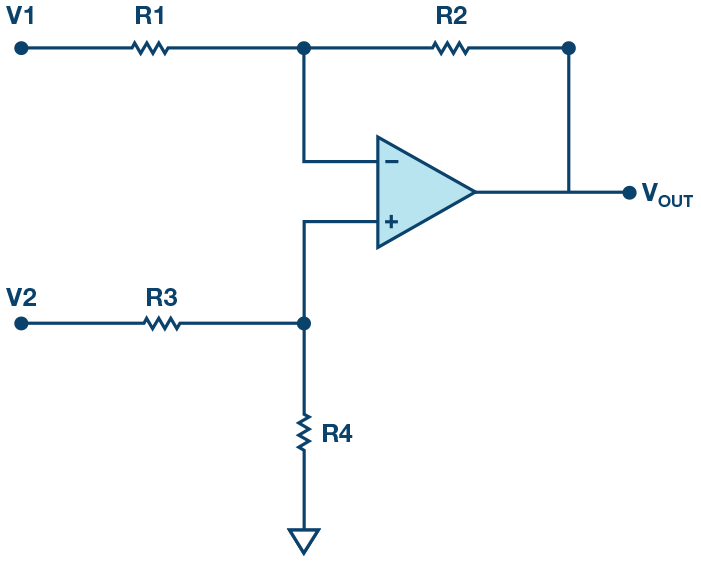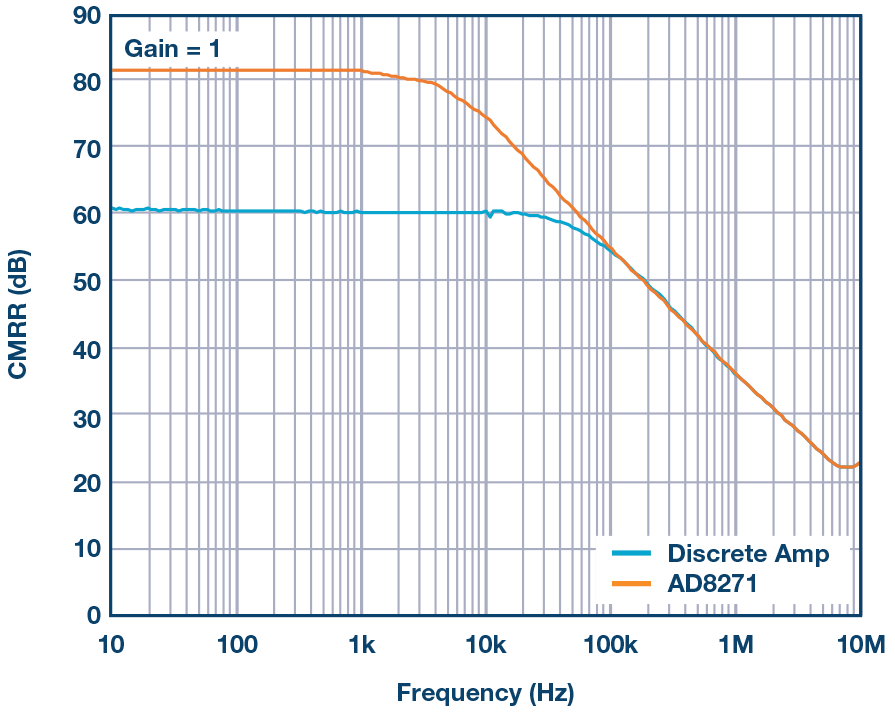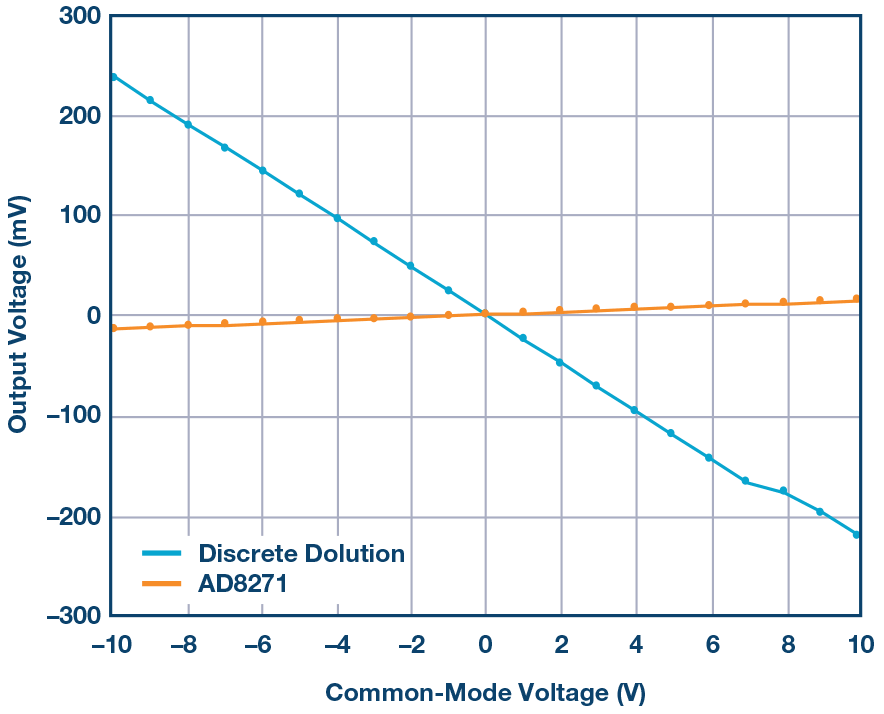# Discrete Difference Amplifier vs. an Integrated Solution

### QUESTION:

Why Pay More for Less?

### Answer:

The classic discrete difference amplifier design is quite simple. What can be complicated about an op amp and a four resistor network?

However, the performance of this circuit may not be as great as the designers would like. Based on actual production designs, this article shows some of the disadvantages encountered with discrete resistors, including gain accuracy, gain drift, ac common-mode rejection (CMR), and offset drift.

The classic four resistor difference amplifier, shown in Figure 1.Figure 1. The classic discrete difference amplifier.

The transfer function of this amplifier is:

With R1 = R3 and R2 = R4, Equation 1 simplifies to

This simplification can be a quick way to approximate the expected signal, but those resistors are never exactly equal. In addition, the resistors usually have low accuracy and a high temperature coefficient, contributing significant errors to the circuit.

For example, using a good op amp and standard 1%, 100 ppm/°C gain-setting resistors, the initial gain error can be up to 2% and may vary by up to 200 ppm/°C. One solution to this issue would be to use monolithic resistor networks for precise gain setting, but those are bulky and expensive. In addition to the low accuracy and significant drift over temperature, most discrete differential op amp circuits have poor CMR and an input voltage range smaller than the power supply voltage. Also, monolithic instrumentation amplifiers (in-amps) will have a gain drift because the internal resistor network of the pre-amps does not match with the external gain setting resistor going to the RG pin.

The best solution to all of these issues is to use a difference amplifier with internal gain setting resistors, such as the AD8271. Typically, these products consist of a high precision, low distortion op amp and several trimmed resistors. These resistors can be connected to create a wide variety of amplifier configurations, including difference, noninverting, and inverting configurations. The resistors on the chip can be connected in parallel for a wider range of options. Using the on-chip resistors provides the designer with several advantages over a discrete design.

Much of the dc performance of op amp circuits depends on the accuracy of the surrounding resistors. Those internal resistors are laid out to be tightly matched, and are laser trimmed and tested for their matching accuracy. Because of this, there is a guarantee of high accuracy for specifications such as gain drift, common-mode rejection, and gain error. The circuit in Figure 1, when integrated, can provide 0.1% gain accuracy with less than 10 ppm/°C gain drift, as seen in Figure 2.Figure 2. Gain error vs. temperature comparison—AD8271 vs. discrete solution.

### AC Performance

The circuit size is much smaller in an integrated circuit than on a printed circuit board (PCB), so the corresponding parasitic factors are also smaller and therefore help the ac performance. For example, the positive and negative input terminals of the AD8271 op amp are not pinned out intentionally. By not connecting these nodes to the traces on the PCB, the capacitance remains low, resulting in both improved loop stability and common-mode rejection over frequency. See Figure 3 for the performance comparison.Figure 3. CMRR vs. frequency comparison—AD8271 vs. discrete solution CMRR.

An important function of the difference amplifier is to reject signals that are common to both inputs. Referring to Figure 1, if the resistors R1 through R4 are not perfectly matched (or if R1, R2 and R3, R4 are not ratio matched when the gain is greater than 1), part of the common-mode voltage will be amplified by the difference amplifier and appear at VOUT as a valid difference between V1 and V2 that cannot be distinguished from a real signal. If the resistors are not perfect, part of the common-mode voltage will be amplified by the difference amplifier and appear at VOUT as a valid difference between V1 and V2 that cannot be distinguished from a real signal. The ability of the difference amplifier to reject this is called common-mode rejection. This can be expressed as common-mode rejection ratio (CMRR) or converted to decibels (dB). With the discrete solution, the resistors are not as well matched as the laser trimmed ones within the integrated solution, as seen by the output voltage vs. CMV plot in Figure 4.Figure 4. Output voltage vs. common-mode voltage—AD8271 vs. discrete solution.

The CMRR, assuming a perfect op amp, is:

where Ad is the gain of the difference amplifier and t is the resistor tolerance. Thus, with unity gain and 1% resistors, the CMRR is 50 V/V, or about 34 dB; with 0.1% resistors, the CMRR increases to 54 dB. Even given a perfect op amp with infinite common-mode rejection, the overall CMRR is limited by resistor matching. Some low cost op amps have a minimum CMRR in the 60 dB to 70 dB range, making the errors worse.

### Low Tolerance Resistors

While amplifiers usually perform well inside of their specified operating temperature range, the temperature coefficient of the external discrete resistors must be taken into account. In the case of an amplifier with integrated resistors, the resistors can be drift trimmed and matched. The layout usually has the resistors close together, so that they all drift together, thus reducing their offset temperature coefficient. In the discrete case, the resistors are spread out further around the PCB and are not as well matched as the integrated case, producing a worse offset temperature coefficient, as shown in Figure 5.Figure 5. System offset vs. temperature—AD8271 vs. discrete solution.

The four resistor difference amplifier—whether discrete or monolithic—is widely used. With only one part, rather than several discrete components, placed on the PCB, the board can be built more quickly, efficiently, and with significant area savings.

To achieve a solid, production-worthy design, carefully consider noise gain, input voltage range, and a CMR of 80 dB or better. These resistors are also manufactured from the same low drift, thin film material, so their ratio match over temperature is excellent.

### Conclusion

It is easy to see the difference between amplifiers with internal gain setting resistors and discrete difference amplifiers.